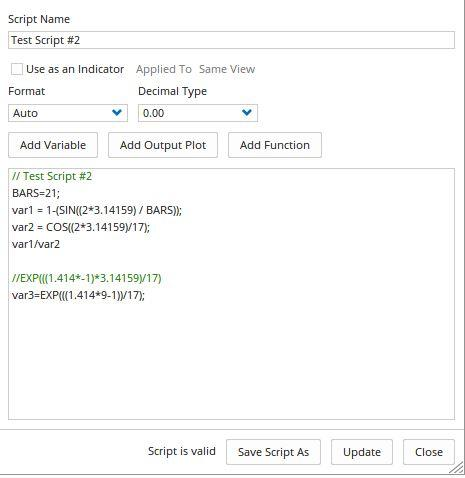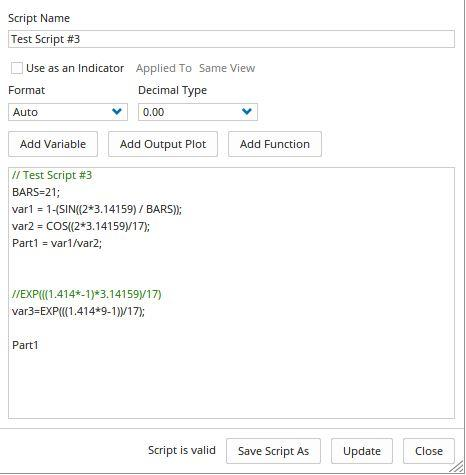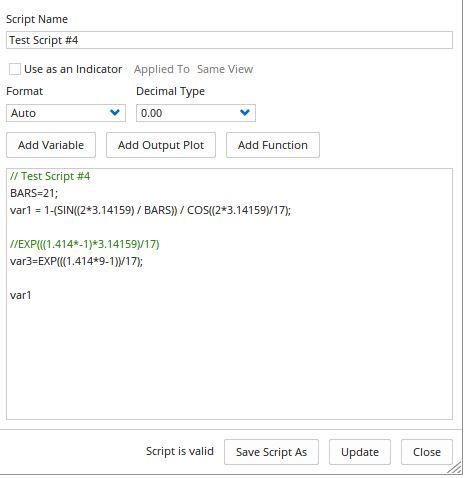Select Page

# Enter Parameters

Optuma Forums Optuma Scripting Enter Parameters

Viewing 9 posts - 1 through 9 (of 9 total)
• Author
Posts
• #51111

Hi,

I am trying to write a script for an indicator but have some problems in doing so. I want therefore ask you for some assistance.

As you can see from the screen shot “test script #1.png” all seems to be right. BUT I want change the numbers “21” and “17” with changeable parameters so that I can change the values latter on as I want.

But when I change “21” with “BARS=21” I get an error message (see screen shot “test script #2.png”.

With the code “var1 / var2” I have also a problem. Since I have to use the division result of “var1 / var2” later on in the formula I have tried to write it as “Part1 = var1 / var2” so that I had only use “Part1” as a reference later on in the formula but this approach resulted also in an error message (see screen shot “test script #3.png”).

Writing the formula in one line as “var1” resulted also in an error message (see screen shot “test script #4.png”).

I hope it is possible for you to give me some advise how can I solve this issue.

Thanks a lot,
Thomas

###### Attachments:
#51131

Hi Thomas,

Can you please copy and paste the script(s) you are using into the thread directly, rather than using a screen image.  This makes sure we’re working with the identical script you are when reviewing it on our own systems here.

#51135

Hi Thomas,

Q1: Changing parameters within a Script (test script #1.png): As far as I’m aware there is no way to change parameters within Scripts when the script is being run or displayed, ie as an external user input once applied to a chart. Any change in values must be made within the Script before execution.

Q2: Test Script #2.png: “Bars=21” is not correct syntactically. The divisor must be a number or a defined variable. You can amend the script to read:

// Test Script #3

BARS=21;

var1 = 1-(SIN((2*3.14159) / BARS));

var2 = COS((2*3.14159)/17);

var1/var2

//EXP(((1.414*-1)*3.14159)/17)

var3=EXP(((1.414*9-1))/17);Q3: Test Script #3.png: The are 2 syntactical issues with this script:

• The line Part1 should end with a “;” and “Part1”, the result, should appear on a new line
• It is recommended as good practice that the output of a Script, in this case “Par1”, be the last code line of the Script

so the code becomes:

// Test Script #3

BARS=21;

var1 = 1-(SIN((2*3.14159) / BARS));

var2 = COS((2*3.14159)/17);

Part1 = var1/var2;

//EXP(((1.414*-1)*3.14159)/17)

var3=EXP(((1.414*9-1))/17);

Part1Note also that all code lines (except comments) must end with a “;”, except the script’s final result line.

Q4: Test Script #3.png: The Script does not has a result line, ie all code lines end with “;”. The Script result line does not have a “;”, so the Script becomes:

// Test Script #4

BARS=21;

var1 = 1-(SIN((2*3.14159) / BARS)) / COS((2*3.14159)/17);

//EXP(((1.414*-1)*3.14159)/17)

var3=EXP(((1.414*9-1))/17);

var1Also, to make it easier for those reviewing and commenting, might I suggest you insert the screenshots in the body of the post so they can be seen in context, along with the code so it can be copied, so commentators don’t have to retype your code to test it.

Cheer

Trevor#51151

Hi all,

thank you very much for your help, especially Trevor. As requested I now copy the script into the mail. I was not familiar with that procedure, sorry.

I am trying to write a script for an indicator but have some problems in doing so. I want therefore ask you for some assistance.

As you can see from the screen shot “test script #1.png” all seems to be right. BUT I want change the numbers “21” and “17” with changeable parameters so that I can change the values latter on as I want.

//test script #1

// (1 – (SIN((2*3.14159) / 21))) / COS((2*3.14159) / 21)

var1 = 1-(SIN((2*3.14159) / 21));
var2 = COS((2*3.14159) / 21);
var1 / var2

//EXP(((1.414*-1)*3.14159)/17)
var3 = EXP(((1.414*9-1)) / 17);

But when I change “21” with “BARS=21” I get an error message (see screen shot “test script #2.png”.

//test script #2

// (1 – (SIN((2*3.14159) / 21))) / COS((2*3.14159) / 21)

var1 = 1-(SIN((2*3.14159) / BARS=21));
var2 = COS((2*3.14159) / 21);
var1 / var2

//EXP(((1.414*-1)*3.14159)/17)
var3 = EXP(((1.414*9-1)) / 17);

With the code “var1 / var2” I have also a problem. Since I have to use the division result of “var1 / var2” later on in the formula I have tried to write it as “Part1 = var1 / var2” so that I had only use “Part1” as a reference later on in the formula but this approach resulted also in an error message (see screen shot “test script #3.png”).

//test script #3

// (1 – (SIN((2*3.14159) / 21))) / COS((2*3.14159) / 21)

var1 = 1-(SIN((2*3.14159) / 21));
var2 = COS((2*3.14159) / 21);
Part1 = var1 / var2

//EXP(((1.414*-1)*3.14159)/17)
var3 = EXP(((1.414*9-1)) / 17);

Writing the formula in one line as “var1” resulted also in an error message (see screen shot “test script #4.png”).

//test script #4

// (1 – (SIN((2*3.14159) / 21))) / COS((2*3.14159) / 21)

var1 = (1-(SIN((2*3.14159) / 21))) / COS((2*3.14159) / 21);

//EXP(((1.414*-1)*3.14159)/17)
var3 = EXP(((1.414*9-1)) / 17);

I hope it is possible for you to give me some advise how can I solve this issue.

Thanks a lot,
Thomas

#51155

Hi Thomas,

I addressed each of the issues you raised in my response above.

Cheers

Trevor

#51159

Hi Trevor,

I think I need again your help. I have now developed the script further and am encounter again a problem.

Here is my current version:

BARS1=21;

BARS2=17;

var1 = (1-(SIN((2*3.14159) / BARS1))) / (COS((2*3.14159) / BARS1));

var2  =  (0.5 * (1 + var1) * (Close() – Close(1))) + (var1 * var2(1));

var3 = EXP(((1.414*9-1)) / BARS2);

var4 = 2 * var3 * (COS((1.414 * 3.14259) / BARS2));

var5 = (var3 * -1) * var3;

var6 =  1 – var4 – var5;

var7 = (var6 * ((var2 + var2(1)) / 2)) + (var4 * var7(1)) + (var5 * var7(2));

var7

Until var7 all has worked fine but var7 resulted an error message.

Let me explain what var7 should do:

Add today’s value of var2 to yesterday’s value of var2, divided this by 2 and multiply the product with var6

Plus the result of today’s value of var4 times yesterday’s value of var7

Plus the result of today’s value of var5 times the day before yesterday’s value of var7

It seems that yesterday’s values and the day before yesterday’s value causing the problems.

I have an Excel file with the formulas and try to script the code in Opatuma. This Excel calculations are working fine.

In the program “TradeStation” the code looks like this:

Inputs:

Duration(40);

Vars:

alpha1(0),

HP(0),

a1(0),

b1(0),

c1(0),

c2(0),

c3(0),

Filt(0),

count(0),

Wave(0),

Pwr(0);

alpha1 = (1 – Sine (360 / Duration)) / Cosine(360 / Duration);

HP = .5*(1 + alpha1)*(Close – Close) + alpha1*HP;

a1 = expvalue(-1.414*3.14159 / 10);

b1 = 2*a1*Cosine(1.414*180 / 10);

c2 = b1;

c3 = -a1*a1;

c1 = 1 – c2 – c3;

Filt = c1*(HP + HP) / 2 + c2*Filt + c3*Filt;

Wave = (Filt + Filt + Filt) / 3;

Pwr = (Filt*Filt + Filt*Filt + Filt*Filt) / 3;

Wave = Wave / SquareRoot(Pwr);

Plot1(Wave);

I am currently at “stage” – Filt = c1*(HP + HP) / 2 + c2*Filt + c3*Filt;

Perhaps this helps.

Thanks again

Thomas

#51163

Hi Thomas,

The first issue I find with your Script is in the var2, because when I reduce your script to:

// Test script #5

BARS1=21;

BARS2=17;

var1 = (1-(SIN((2*3.14159) / BARS1))) / (COS((2*3.14159) / BARS1));

var2 = (0.5 * (1 + var1) * (Close(0) – Close(1)))+ (var1 * var2(1));

var2

I get the following:This shows that var2 is not working, because, I believe, it is calling itself with your required OFFSET of 1, by the variable var2(1). By the way, if you are wanting an OFFSET from a variable you need to use square brackets “[]”, which would mean that line would read:

var2 = (0.5 * (1 + var1) * (Close(0) – Close(1)))+ (var1 * var2);

but that too, will show a scripting error as var2 is calling itself.

You will run into the same issue with var7, as it too is calling itself a couple of times within the variable calculation.

You will need to rearrange your formulae so that there is no recursive calling of variables.

Cheers

Trevor#51175

Hi,

a question to all Optuma Script experts.

Trevor suggested me I should rearrange my script because some variables are calling itself.

My question now is: “Is there at all a possibility in Optuma to rearrange my script in a way that it works or is it simply impossible?”

If it is possible please give me some hints how I can do it.

Many thanks,

Thomas

#51419

Hi,

I have once again a question concerning the scripting of my indicator.

Is there a possibility to use the Pascal Programming module to create a custom tool of my above mentioned indicator?

Thanks,

Thomas

Viewing 9 posts - 1 through 9 (of 9 total)

You must be logged in to reply to this topic.

### Pin It on Pinterest

By continuing to use the site, you agree to the use of cookies. more information

The cookie settings on this website are set to "allow cookies" to give you the best browsing experience possible. If you continue to use this website without changing your cookie settings or you click "Accept" below then you are consenting to this.

Close